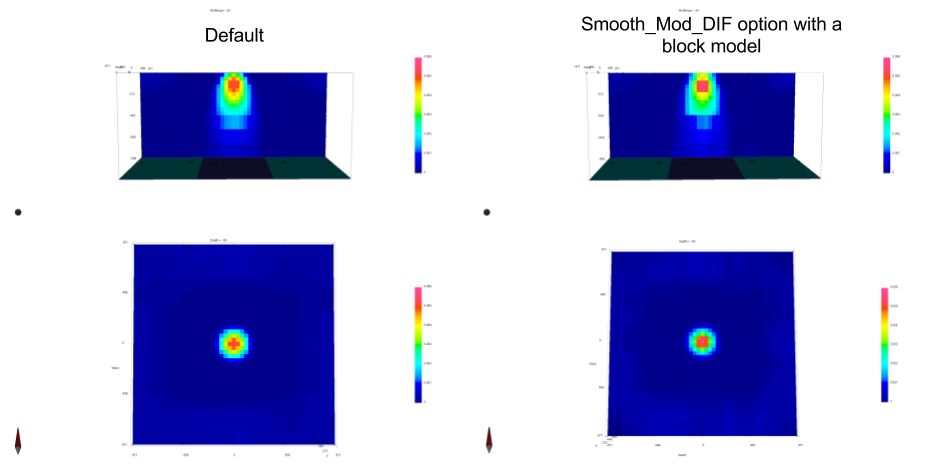# 6.8. The SMOOTH_MOD_DIF or SMOOTH_MOD options¶

(1)$\begin{split}\phi_m(\mathbf{m}) = &{\alpha_s} ||\mathbf{W_s}\;\mathbf{R}_s(\mathbf{m}-\mathbf{m}_{ref})||_2^2 +\\ &{\alpha_x} ||\mathbf{W_x}\;\mathbf{R}_x \; \mathbf{G}_x(\mathbf{m}-\mathbf{\color{blue}m}_{\color{blue}ref})||_2^2 +\\ &{\alpha_y} ||\mathbf{W_y}\;\mathbf{R}_y \; \mathbf{G}_y(\mathbf{m}-\mathbf{\color{blue}m}_{\color{blue}ref})||_2^2 +\\ &{\alpha_z} ||\mathbf{W_z}\;\mathbf{R}_z \; \mathbf{G}_z(\mathbf{m}-\mathbf{\color{blue}m}_{\color{blue}ref})||_2^2\end{split}$

Introduced in v6 of the gravity and magnetics codes, this toggle controls whether the reference model is used in the gradient terms of the model objective function.

If the reference model is used in the gradient terms (SMOOTH_MOD_DIF), then sharp boundaries that are present in the reference model will be preserved. If not used (SMOOTH_MOD), the difference to the gradient model will still be minimised, but the boundary is allowed to have a smooth transition.